EP. 006 we welcome Electrical Engineer Kelvin Abraham to discuss his unifying theory for everything (mass-Energy-Matter) – Tetryonics, and how using Tetryonics to build accurate visual representations of atoms will advance us into a new age of prosperity.

Before we brought Kelvin on the show, Alex and I had a chance to read out a few listener communiqués.

During the conversation, we discuss how Tetryonics works in providing a visual model for atoms, how Tetryonics was come about, problems that were solved in the process of developing the unified theory, and practical applications in providing never ending energy for all of man kind.

## Tetryonics

Tetryonics is a new geometric theory of mass-ENERGY-Matter
and the forces of motion, stemming from a geometric re-interpretation of what squared numbers are in physics and its application to quantized angular momentum (QAM).

It can now be shown through geometry that the QAM of Planck’s constant is in fact the result of its quantized equilateral ‘triangular’ geometry (and not a vector rotation about a point as has been historically assumed to date).

Tetryonics University:
Kelvin has had his paper on Tetryonics published in the International Journal of Scientific and Research Publications’s May 2014 edition.

http://www.ijsrp.org/research-paper-0514.php?rp=P292642

#### Visual examples of Tetryonics as discussed in this episode: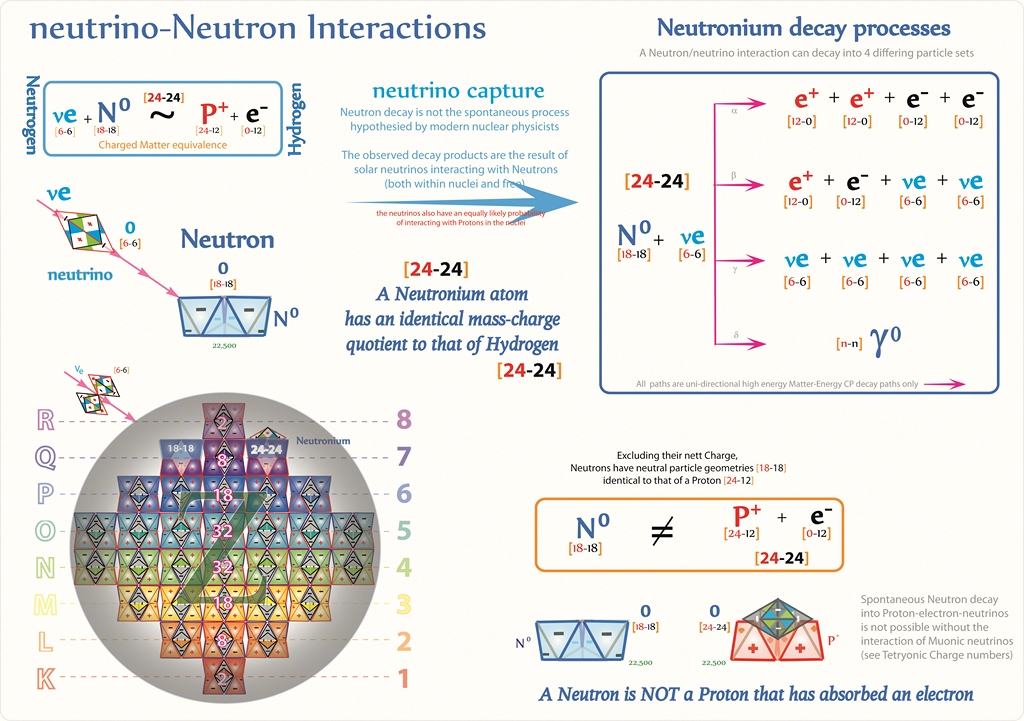The charged geometry of mass-energy-Matter topologies and their Forces of motion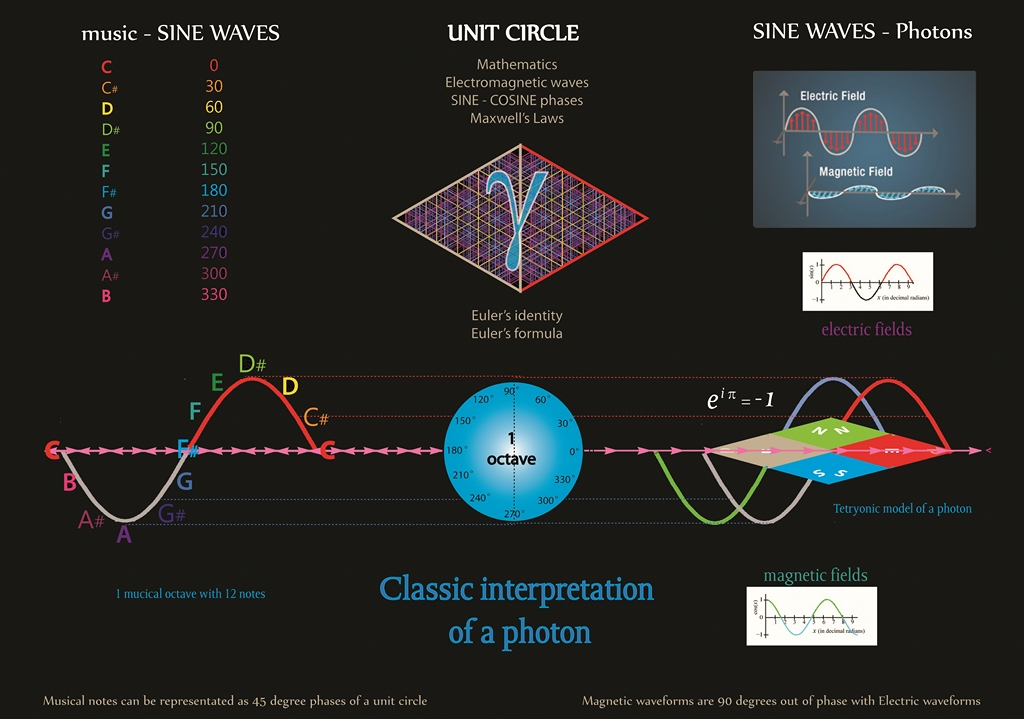The charged geometry of mass-energy-Matter topologies and their Forces of motionThe charged geometry of mass-energy-Matter topologies and their Forces of motion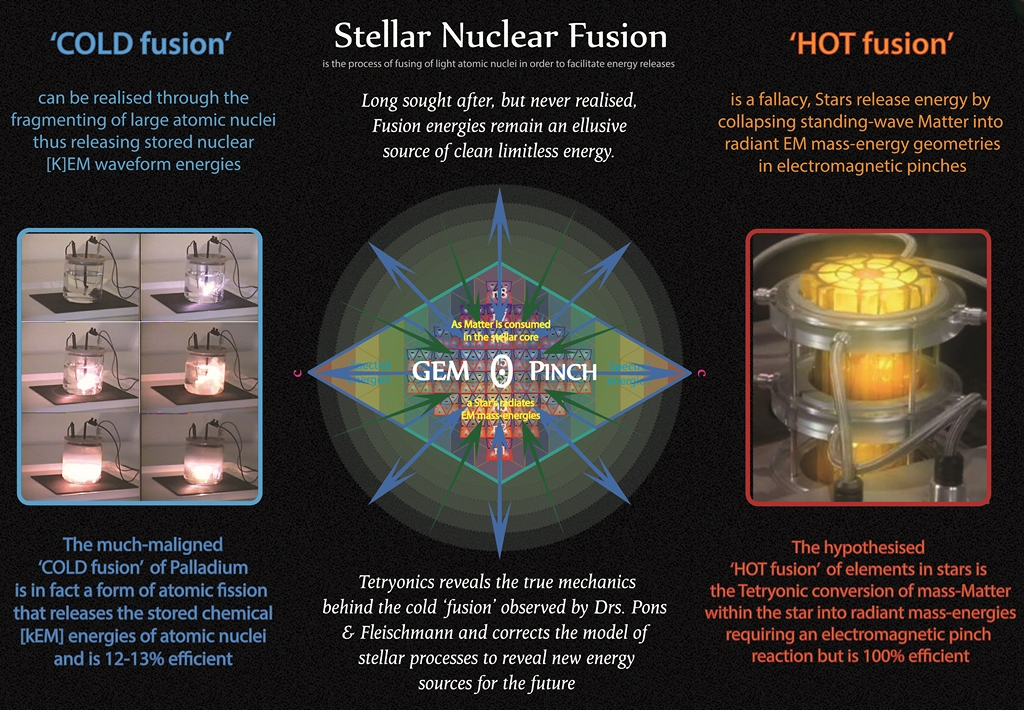The charged geometry of mass-energy-Matter topologies and their Forces of motion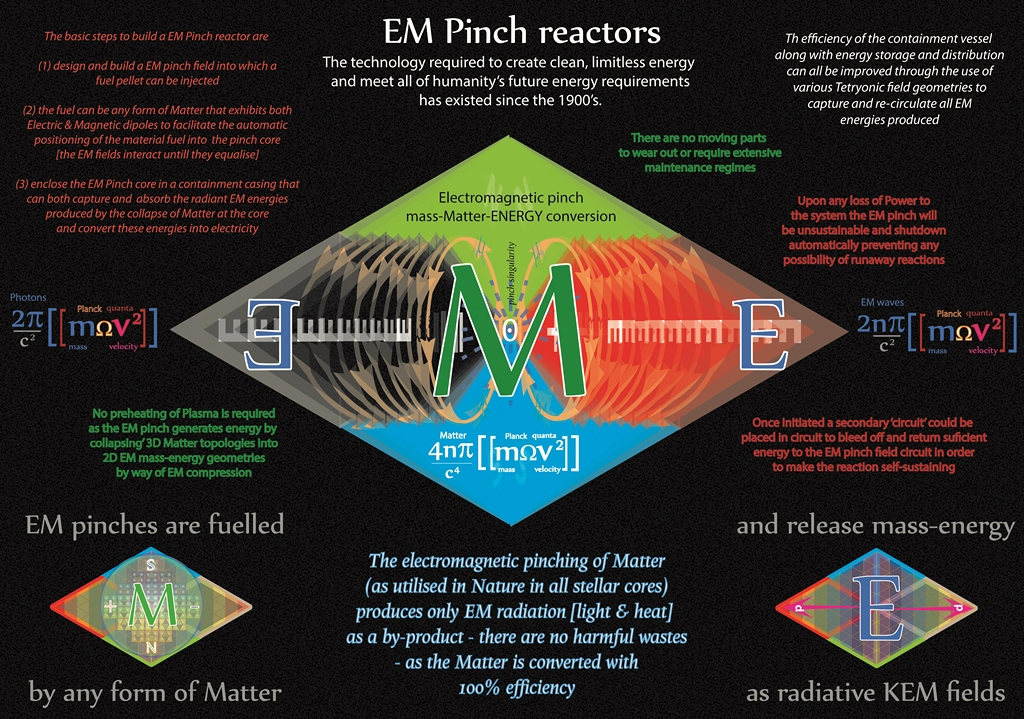The charged geometry of mass-energy-Matter topologies and their Forces of motion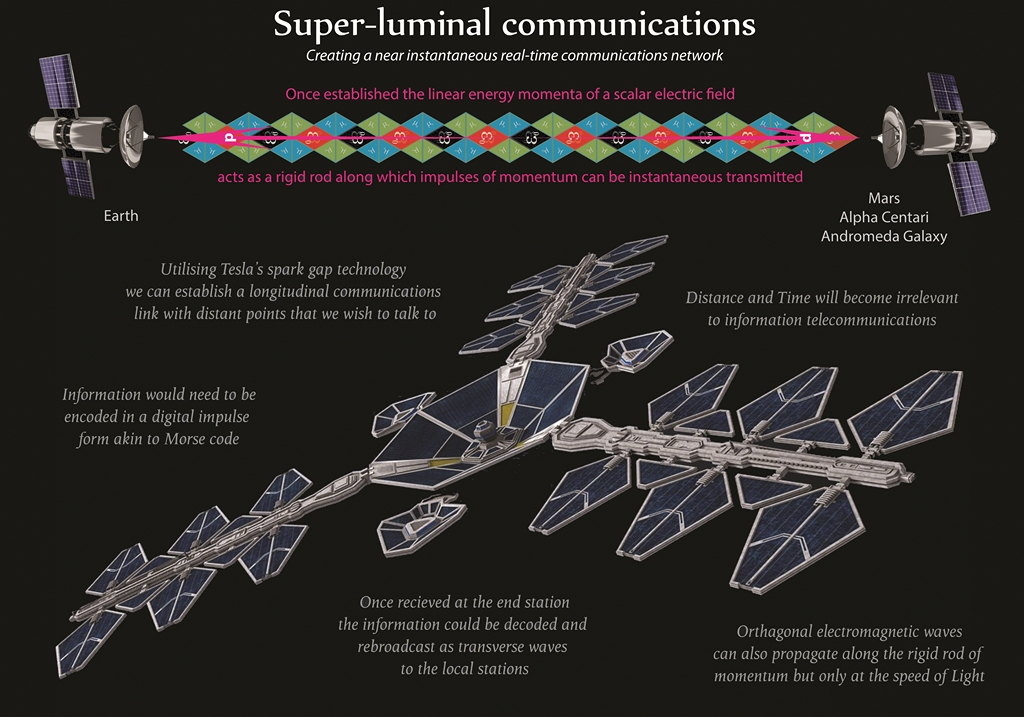The charged geometry of mass-energy-Matter topologies and their Forces of motion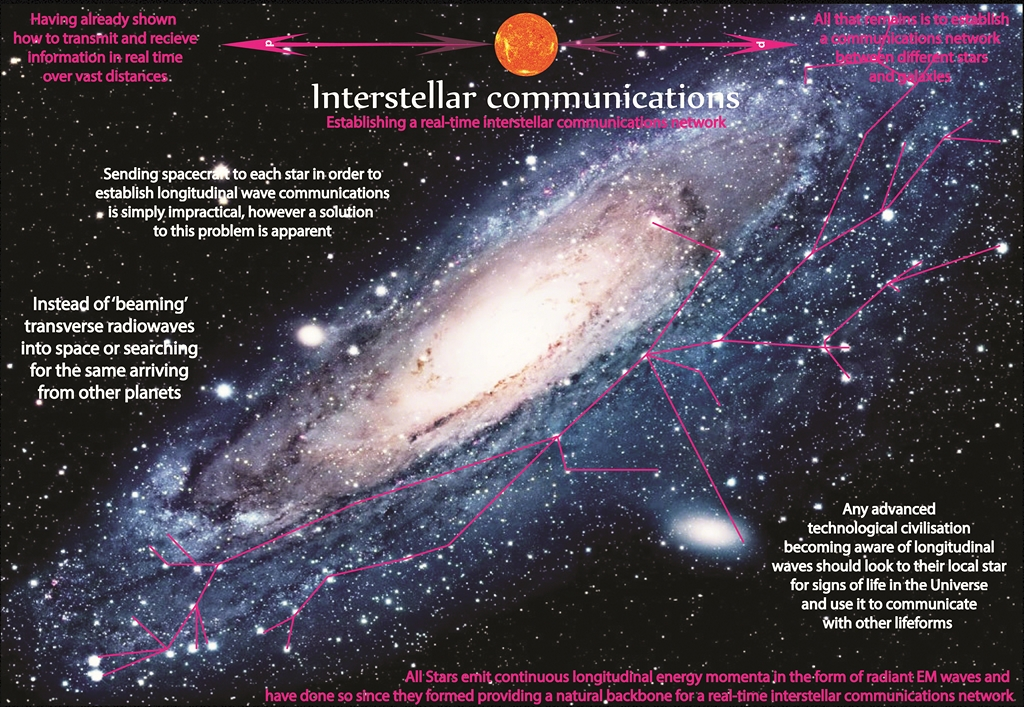The charged geometry of mass-energy-Matter topologies and their Forces of motion